Courses

# Collisions, Motion Of A Group Of Particles Physics Notes | EduRev

## Physics : Collisions, Motion Of A Group Of Particles Physics Notes | EduRev

The document Collisions, Motion Of A Group Of Particles Physics Notes | EduRev is a part of the Physics Course Basic Physics for IIT JAM.
All you need of Physics at this link: Physics

A collision is an encounter between two bodies that alters at least one of their courses. Altering the course of a body requires that a force be applied to it. Thus, each body exerts a force on the other. These forces of interaction may operate at some distance, as do the gravitational and electromagnetic forces, or the bodies may appear to make physical contact. However, even apparent contact between two bodies is only a macroscopic manifestation of microscopic forces that act between atoms some distance apart. There is no fundamental distinction between physical contact and interaction at a distance.
The importance of understanding the mechanics of collisions is obvious to anyone who has ever driven an automobile. In modern physics, however, collisions are important for a different reason. The current understanding of the subatomic particles of which atoms are composed is derived entirely from studying the results of collisions among them. Thus, in modern physics, the description of collisions is a significant part of the understanding of matter. These descriptions are quantum mechanical rather than classical, but they are nevertheless closely based on principles that arise out of classical mechanics.
It is possible in principle to predict the result of a collision using Newton’s second law directly. Suppose that two bodies are going to collide and that F, the force of interaction between them, is known to be a function of r, the distance between them. Then, if it is known that, say, one particle has incident momentum p, the problem is solved if the final momentum p + Δp can be determined. Inverting Newton’s second law, F = dp/dt, the change in momentum is given by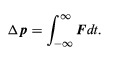(63)

This integral is known as the impulse imparted to the particle. In order to perform the integral, it is necessary to know r at all times so that F may be known at all times. More realistically, Δp is the sum of a series of small steps, such that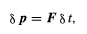(64)

where F depends on the instantaneous distance between the particles. Because p = mv = mdr/dt, the change in r in this step is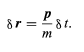(65)

At the next step, there is a new distance, r + δr, giving a new value of the force in equation (64) and a new momentum, p + δp, in equation (65). This method of analyzing collisions is used in numerical calculations on digital computers.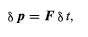(64)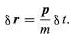(65)

To predict the result of a collision analytically (rather than numerically) it is often most useful to apply conservation laws. In any collision (as in any other phenomenon), energy, momentum, and angular momentum are always conserved. Judicious application of these laws may be extremely useful because they do not depend in any way on the detailed nature of the interaction (i.e., the force as a function of distance).
This point can be illustrated by the following example. A collision is to take place between two bodies of the same mass m. One of the bodies is initially at rest (its momentum is zero). The other has initial momentum p0. After the collision, the body previously at rest has momentum p1, and the body initially in motion has momentum p2. Since momentum is conserved, the total momentum after the collision, p1 + p2, must be equal to the total momentum before the collision, p0; that is,
p0 = p+ p2           (66)

Equation (66) is the equation of a vector triangle, as shown in Figure. However, p1 and p2 are not determined by this condition; they are only constrained by it.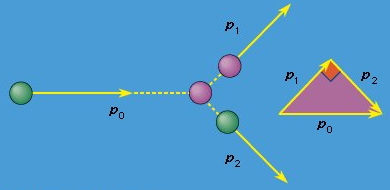Figure: Collision between two particles of equal mass.

Although energy is always conserved, the kinetic energy of the incident body is not always converted entirely into the kinetic energy of the two bodies after the collision. For example, if the bodies are microscopic (say, two identical atoms), the collision may cause one or both to be excited into a state of higher internal energy than it started with. Such an event would leave correspondingly less kinetic energy for the outgoing atoms. In fact, it is precisely by studying the trajectories of outgoing projectiles in collisions like these that physicists are able to determine the possible excited states of microscopic particles.
In a collision between macroscopic objects, some of the kinetic energy is always converted to heat. Heat is the energy of random vibrations of the atoms and molecules that constitute the bodies. However, if the amount of heat is negligible compared to the initial kinetic energy, it may be ignored. Such a collision is said to be elastic.
Suppose the collision described above between two bodies, each of mass m, is between billiard balls, and suppose it is elastic (a reasonably good approximation of real billiard balls). The kinetic energy of the incident ball is then equal to the sum of the kinetic energies of the outgoing balls. According to equation (3), the kinetic energy of a moving object is given by K = 1/2mv2, where v is the speed of the ball (technically, the energy associated with the fact that the ball is rolling as well as translating is ignored here; see below Rotation about a moving axis). Equation (3) may be written in a particularly useful form by recognizing that since p = mv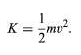(3)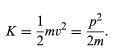(67)

Then the conservation of kinetic energy may be written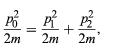(68)

or, canceling the factors 2m,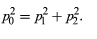(69)

Comparing this result with equation (66) shows that the vector triangle is pythagorean; p1 and p2 are perpendicular. This result is well known to all experienced pool players. Notice that it was possible to arrive at this result without any knowledge of the forces that act when billiard balls collide.

Offer running on EduRev: Apply code STAYHOME200 to get INR 200 off on our premium plan EduRev Infinity!

,

,

,

,

,

,

,

,

,

,

,

,

,

,

,

,

,

,

,

,

,

,

,

,

;